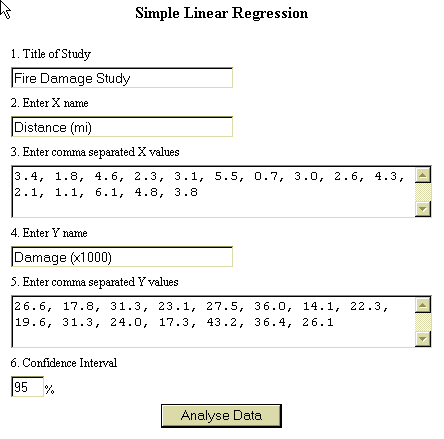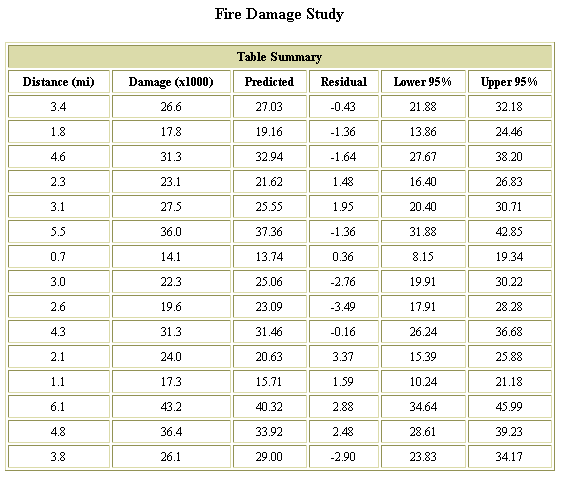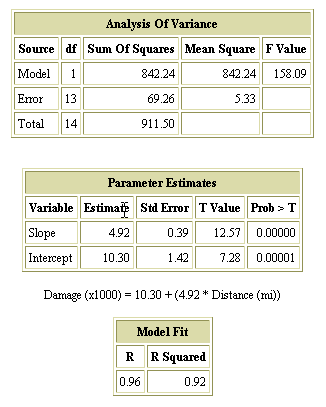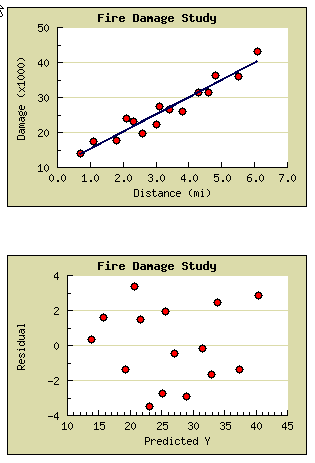Search

Useful Lists

Partners

Online Manuals

Simple linear regression with PHP: Part 2
By Paul Meagher - 2004-05-21 Page:  1 2 3 4 5 6 7 8 9

## Fire damage study

To demonstrate how to use the data-exploration tool, I will use data from a hypothetical fire damage study. This study correlates the amount of fire damage in major residential fires to their distance from the nearest fire station. Insurance companies, for example, would be interested in studying this relationship for the purpose of determining premiums.

The data for the study are shown in the input screen in Figure 1.

Figure 1. Input screen shows study dataWhen the data is submitted, it is analyzed and the results of those analyses are displayed. The first result set to display is the Table summary, shown in Figure 2.

Figure 2. Table summary is first set of results displayedThe table summary displays, in tabular form, the input data along with other columns indicating the predicted Y value for the observed X value, the difference between the predicted and observed Y values, and the lower and upper confidence intervals for the predicted Y value.

Figure 3 shows three higher-level summaries of the data that come after the table summary.

Figure 3. Three high-level data summaries follow table summaryThe Analysis of variance table shows how the variance of the Y scores is partitioned into two main sources of variance -- the variance accounted for by the model (see the Model row) and the variance unaccounted for (see the Error row). A large F value tells you that the linear model captures most of the variance in your Y measurements. This table becomes even more useful in Multiple Regression contexts in which each independent variable has a row in the table.

The Parameter estimates table shows the estimated Y Intercept and Slope. Each row includes a T value and the probability of observing a T value that extreme (see the Prob > T column). The Prob > T for the Slope can be used to reject a linear model.

If the probability of the T value is greater then 0.05, or some similarly low probability, then you can reject the null hypothesis because a value that extreme has a low likelihood of being observed by chance. Otherwise you must retain the null hypothesis.

In the fire damage study, the probability of obtaining a T value of 12.57 by chance is less than 0.00000. This means that a linear model is a useful predictor of Y values (better than the mean of the Y values) for the range of X values observed in the study.

The final report displays correlation coefficients or R values. They can be used to assess how well your linear model fits the data. High R values indicate a good fit.

Each summary report provides answers to different analytic questions that you might have about the relationship of your linear model to the data. Consult textbooks by Hamilton, Neter, or Pedhauzeur for more advanced treatment of regression analysis (see Resources).

The final report elements to display are the scatter and line plots of the data, as seen in Figure 4.

Figure 4. Final report elements -- scatter and line plotsMost people are familiar with interpreting line graphs such as the top graphic in this series, so I won't comment except to say that the JPGraph library produces high-quality scientific plots for the Web. It also does the right thing when you feed in your scatter and line data.

The second plot relates residuals (Observed Y, Predicted Y) to your predicted Y scores. This is an example of a graph used by proponents of Exploratory Data Analysis (EDA) to help maximize the analyst's ability to detect and understand patterns in data. This graph can be used by the trained eye to answer questions about:

• Potential outliers or overly influential cases
• Possible curvilinear relation (use Transformation?)
• Non-normal residual distribution
• Non-constant error variance or heteroscedasticity

This data-exploration tool could easily be extended to generate more types of graphs -- histograms, box plots, quartile plots -- that are standard EDA tools.

View Simple linear regression with PHP: Part 2 Discussion

Page:  1 2 3 4 5 6 7 8 9 Next Page: Math library architecture